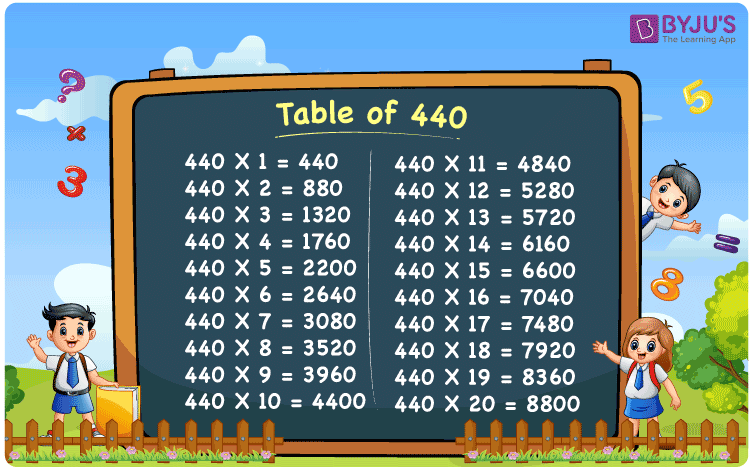Checkout JEE MAINS 2022 Question Paper Analysis : Checkout JEE MAINS 2022 Question Paper Analysis :

# Table of 440

The Multiplication Table for 440 is nothing more than the repetitive addition of the number 440. Repetitive addition is adding the same number multiple times. For example, adding 5 times 440, which is 440 + 440 + 440 + 440 + 440 is the same as 440 x 5 and that equals 2200. It would be easy to remember the table for 44 and use the same for 440 as well, but to end the multiples with a 0. For any large number multiplication table, use the Multiplication Calculator.

## Table of 440 Chart## What is the 440 Times Table?

In the table of 440, we are repeatedly adding 440 multiple times. And the table below gives a repetitive addition 10 times for the number 440.

 440 × 1 = 440 440 440 × 2 = 880 440 + 440 = 880 440 × 3 = 1320 440 + 440 + 440 = 1320 440 × 4 = 1760 440 + 440 + 440 + 440 = 1760 440 × 5 = 2200 440 + 440 + 440 + 440 + 440 = 2200 440 × 6 = 2640 440 + 440 + 440 + 440 + 440 + 440 = 2640 440 × 7 = 3080 440 + 440 + 440 + 440 + 440 + 440 + 440 = 3080 440 × 8 = 3520 440 + 440 + 440 + 440 + 440 + 440 + 440 + 440 = 3520 440 × 9 = 3960 440 + 440 + 440 + 440 + 440 + 440 + 440 + 440 + 440 = 3960 440 × 10 = 4400 440 + 440 + 440 + 440 + 440 + 440 + 440 + 440 + 440 + 440 = 4400

## Multiplication Table of 440

Given below is the multiplication table of 440 being done 20 times. The below table helps you with multiplication problems that require the tables of 440.

 440 × 1 = 440 440 × 2 = 880 440 × 3 = 1320 440 × 4 = 1760 440 × 5 = 2200 440 × 6 = 2640 440 × 7 = 3080 440 × 8 = 3520 440 × 9 = 3960 440 × 10 = 4400 440 × 11 = 4840 440 × 12 = 5280 440 × 13 = 5720 440 × 14 = 6160 440 × 15 = 6600 440 × 16 = 7040 440 × 17 = 7480 440 × 18 = 7920 440 × 19 = 8360 440 × 20 = 8800

## Solved Example on Table of 440

Example:

44 people were distributed Rs. 500. All 44 people returned Rs 60 from their Rs. 500. How much money was actually distributed?

Solution: Rs. 19360

Returned Rs 60

Therefore it is 500 – 60 = Rs 440

Each person got Rs 440. There were 44 people.

So it will be 440 x 44 = 19360

Each received Rs 440, and a total of Rs 19360 was distributed.

## Frequently Asked Questions on Table of 440

### Question 1: What is 15 times 440?

Answer: 15 times 440 is 6600.

### Question 2: What is 4 times 440?

Answer: 4 times 440 is 1760.

### Question 3: Is 440 a perfect square number?

Answer: No. 440 is not a perfect square number. 400 is a perfect square as 20 times 20 is 400.